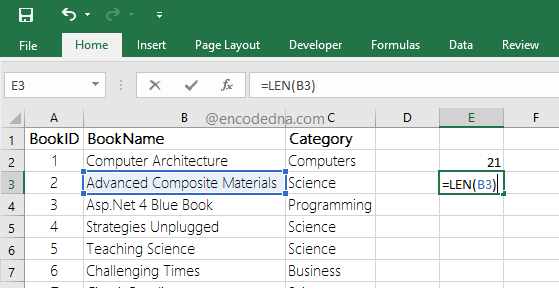﻿ Count Total Number of Characters in a Cell in Excel

# Count Total Number of Characters in a Cell in Excel

You can use the LEN() function in Excel to count the total number of characters in a cell in your worksheet. The LEN (or the length) function takes a parameter (or argument), which can be a string or address of a cell.## LEN Syntax

LEN(text)

How do you use it? Type or enter the = sign in a cell, followed by the function LEN() with a text or a string of values. For example,

=LEN("I am Arun Banik")

It will return 15. Remember, the values are inside two double quotes.

## Count total Characters in a Cell

Now, to count the total characters in a Cell, simply provide the address of the cell as parameter to the function. See the image above.

Let’s assume, I have a value Advanced Computer Materials in cell B3, the formula to get the total characters in the cell would be,

=LEN(B3)

The result will be 28, all characters along with a two spaces.

Note: The LEN will return total number of characters (alphabets, numbers, special characters etc.) including spaces.

## Using LEN with other Functions

The LEN() function can also be used along with other functions. For example,

=SUMPRODUCT(LEN(B2:B10))

Here, I am trying to get the total count of all the characters in the range B2:B10 (again, see the above image).

Yes, if it’s a range of cells for which you want to count the total characters, then you’ll have to use LEN along with SUMPRODUCT function.

## Using LEN with IF function

You can use the LEN with IF function. For example,

=IF(LEN(B8) > 10, "too many characters")

The above formula will check if number of characters in cell B8 is greater than 10, then show a message.

Well, that’s it. Thanks for reading.

Like this Article? Subscribe now, and get all the latest articles and tips, right in your inbox.
Delivered by FeedBurner